## NCERT Solutions for Class 10 Maths Chapter 8 Introduction to Trigonometry Ex 8.2

NCERT Solutions for Class 10 Maths Chapter 8 Introduction to Trigonometry Ex 8.2 are part of NCERT Solutions for Class 10 Maths. Here we have given NCERT Solutions for Class 10 Maths Chapter 8 Introduction to Trigonometry Ex 8.2.

 Board CBSE Textbook NCERT Class Class 10 Subject Maths Chapter Chapter 8 Chapter Name Introduction to Trigonometry Exercise Ex 8.2 Number of Questions Solved 4 Category NCERT Solutions

## NCERT Solutions for Class 10 Maths Chapter 8 Introduction to Trigonometry Ex 8.2

Class 10 Ex 8.2 Question 1.
Evaluate the following: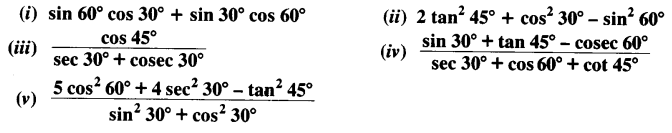Solution: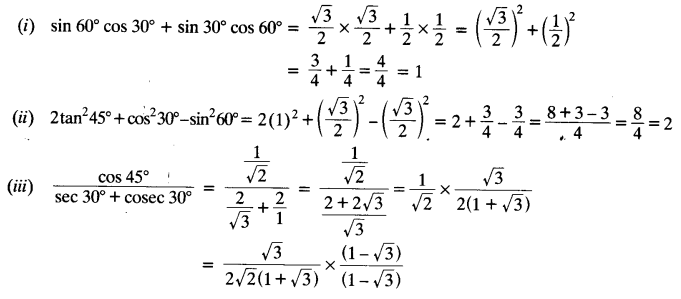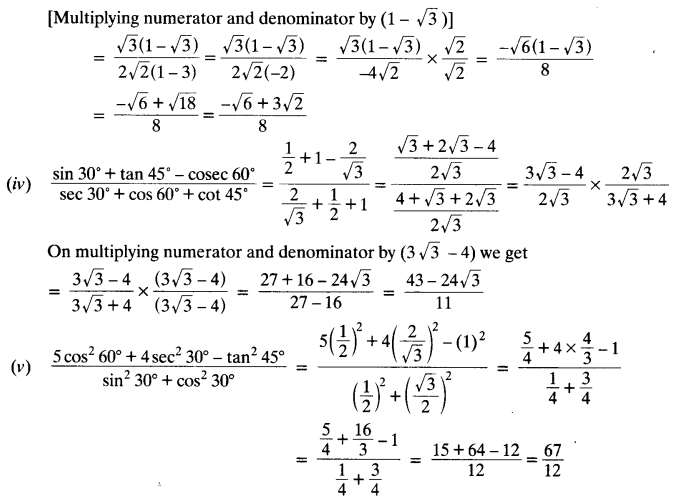Ex 8.2 Class 10 Question 2.
Choose the correct option and justify your choice:Solution: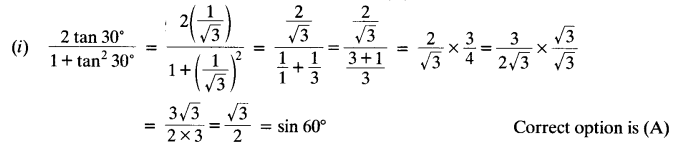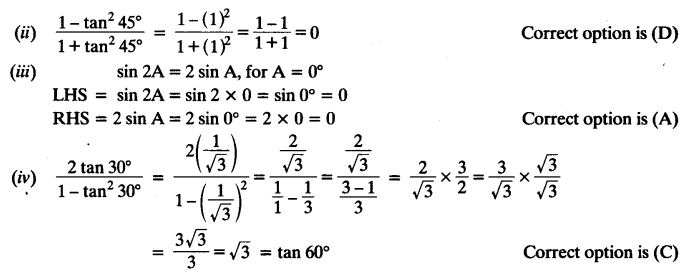Exercise 8.2 Class 10 Question 3.
If tan (A + B) = √3 and tan (A – B) = $$\frac { 1 }{ \surd 3 }$$; 0° < A + B ≤ 90°; A > B, find A and B.
Solution:
tan (A + B) = √3
⇒ tan (A + B) = tan 60°
⇒ A + B = 60° ……(i)
tan (A – B) = $$\frac { 1 }{ \surd 3 }$$
⇒ tan (A – B) = tan 30°
⇒ A – B = 30° ……..(ii)
Adding equation (i) and (ii), we get
2A = 90° ⇒ A = 45°
From (i), 45° + B = 60° ⇒ B = 60° – 45° = 15°
Hence, ∠A = 45°, ∠B = 15°

Exercise 8.2 Class 10 NCERT Solution Question 4.
State whether the following statements are true or false. Justify your answer.
(i) sin (A + B) = sin A + sin B.
(ii) The value of sin θ increases as θ increases.
(iii) The value of cos θ increases as θ increases.
(iv) sin θ = cos θ for all values of θ.
(v) cot A is not defined for A = 0°.
Solution: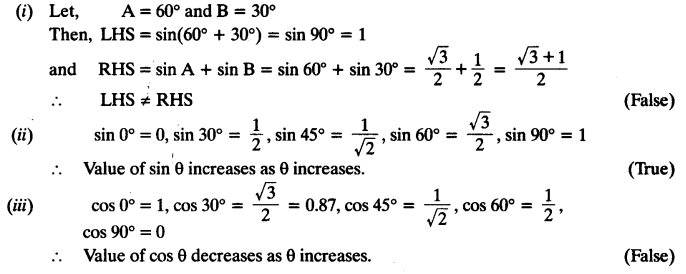We hope the NCERT Solutions for Class 10 Maths Chapter 8 Introduction to Trigonometry Ex 8.2, help you. If you have any query regarding NCERT Solutions for Class 10 Maths Chapter 8 Introduction to Trigonometry Ex 8.2, drop a comment below and we will get back to you at the earliest.